Question

Matlab And Simulation

(a) A DC voltage Vis applied to the microelectromechanical systems (MEMS)misaligned double-plate electrodes shown in Figure Q 1(a). The corresponding electrostatic force in Fd,direction is -epsilon0epsilonrWLV2)/ (2d2)(epsilon0 and epsilonr denote permittivity in vacuum and relative permittivity respectively. W, L, d are width, length, and gap between the two plates respectively). A linear scaling for the voltage is used, i.e. V x l',where I is the linear scale of the electrode. What happens to Fd if all dimensions of the two plates are reduced to 50% of their initial values?

(b) For the three differential equations below describing MEMS dynamic systems, write their transfer functions based on the Laplace Transform.Work out fundamental frequency for each case (neglect the damping effect).

3 \ddot{x}(t)+11 \dot{x}(t)+300 x=3 f(t)

a \dot{x}(t)+25 a x(t)=c f(t) ; \quad \text { a and } c \text { are non-zero constants }

\frac{1}{3} \ddot{x}(t)+27 x(t)=5 f(t)

(c) There is a parallel plate MEMS actuator shown in Figure Q 3(c). The initial gap go is 12 µm, and the area of the plate A is 200 µm x 100 µm. (Note:free space permittivity is 8.85 x 10-12 F/m)

(1) Derive the formula for the electrostatic force F;

(2) Assume a 5 volts potential is applied to the actuator. Calculate F when the top plate is in its initial position.

(3) Derive the equation for Vvs. g and draw a graph showing V vs. g (g varies from 0 to 12 um with 2 µm step size; given spring constant kis 30 N/m). Give a brief explanation about the pull-in voltage.

(d) Describe the working principle of a strain sensor that is made of a piezoresistive material and a piezoelectric material respectively.Verified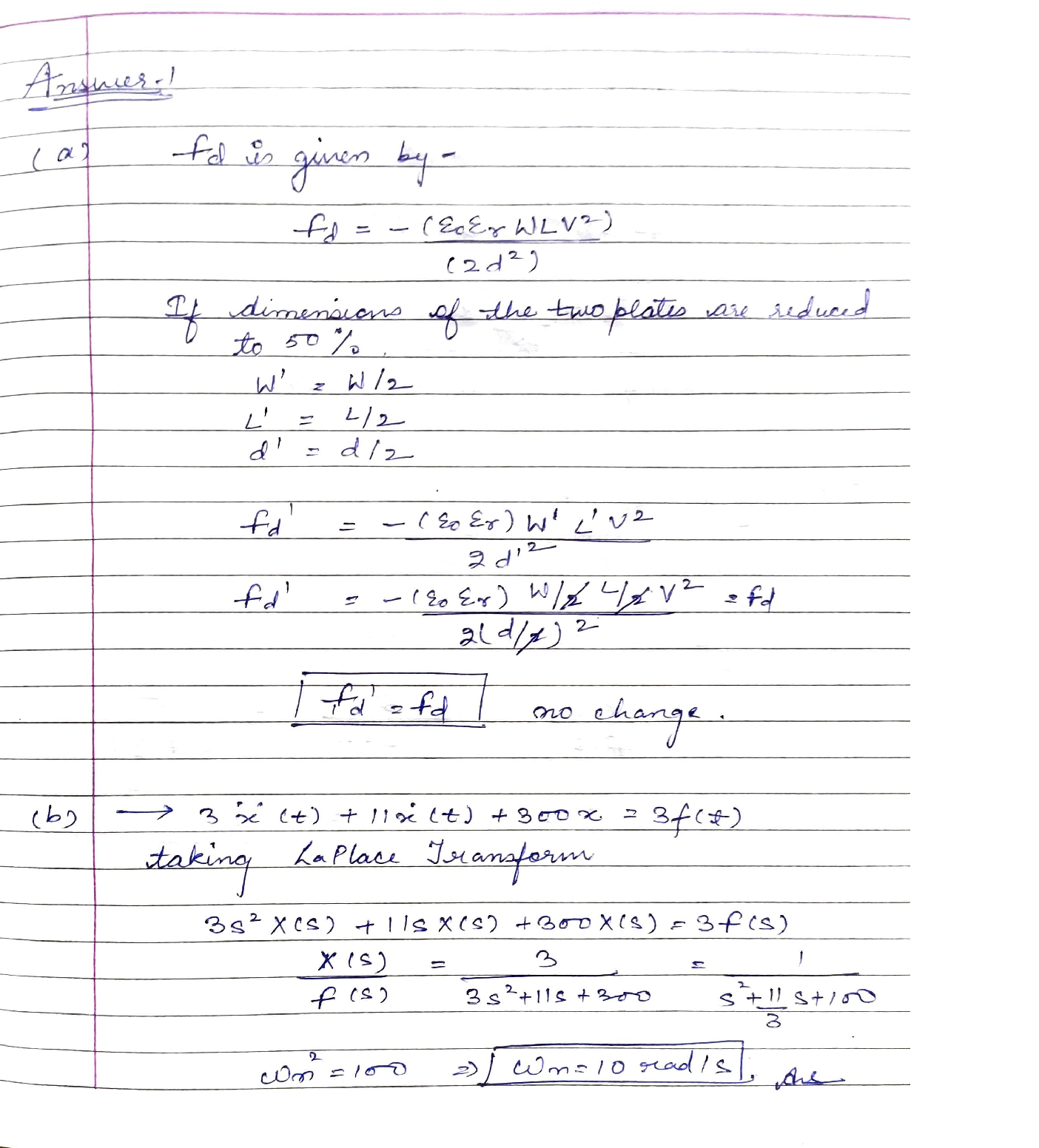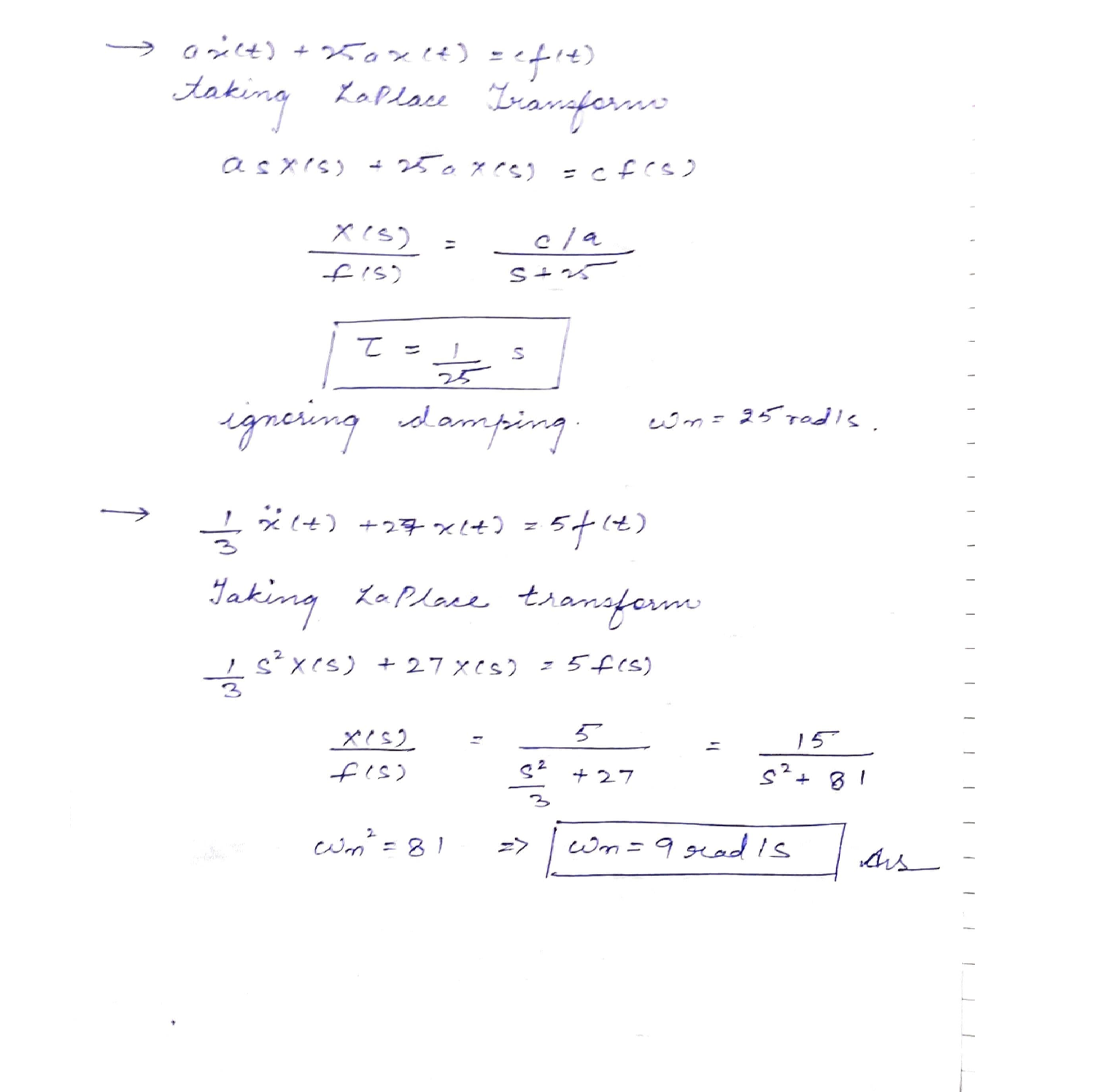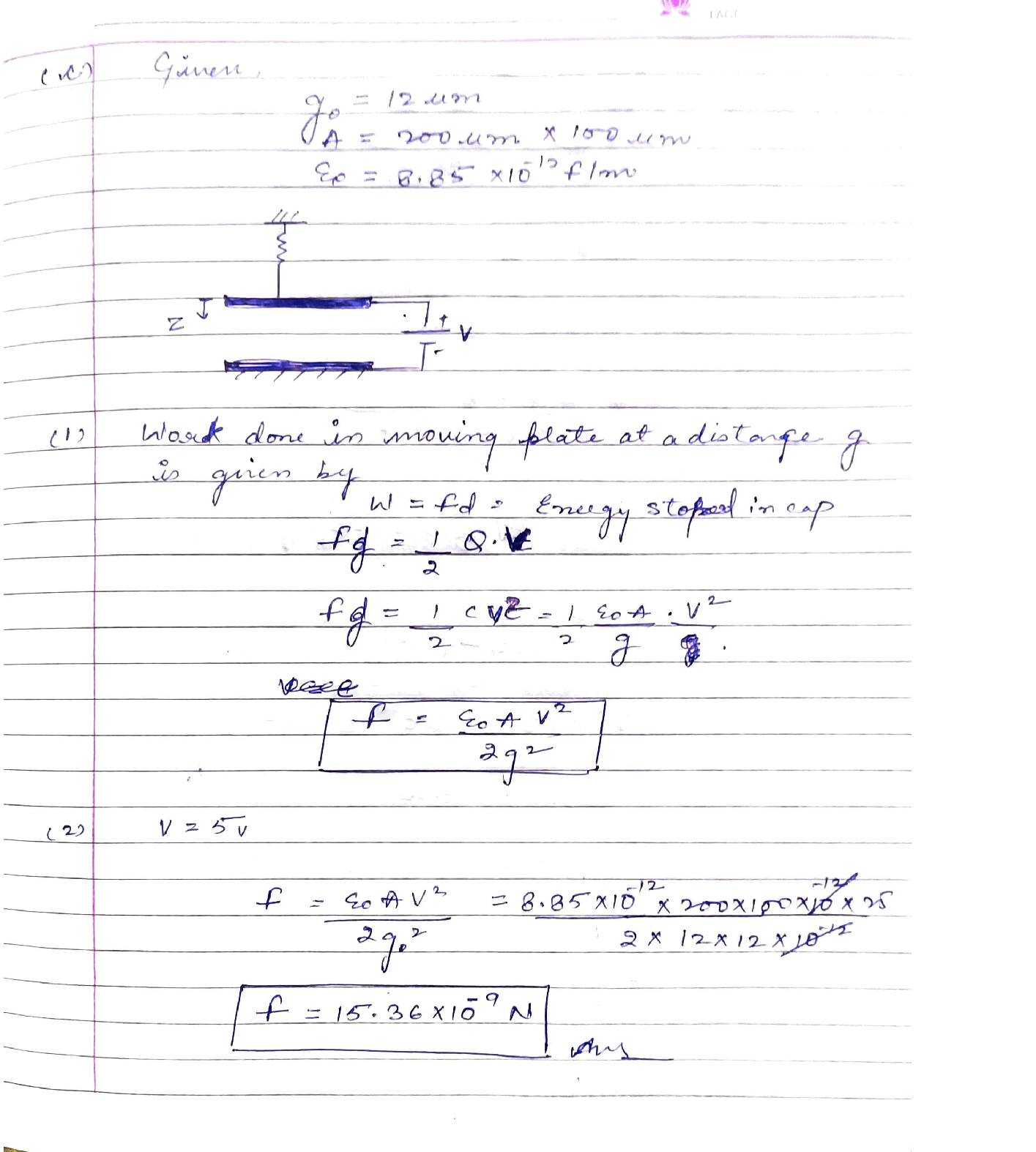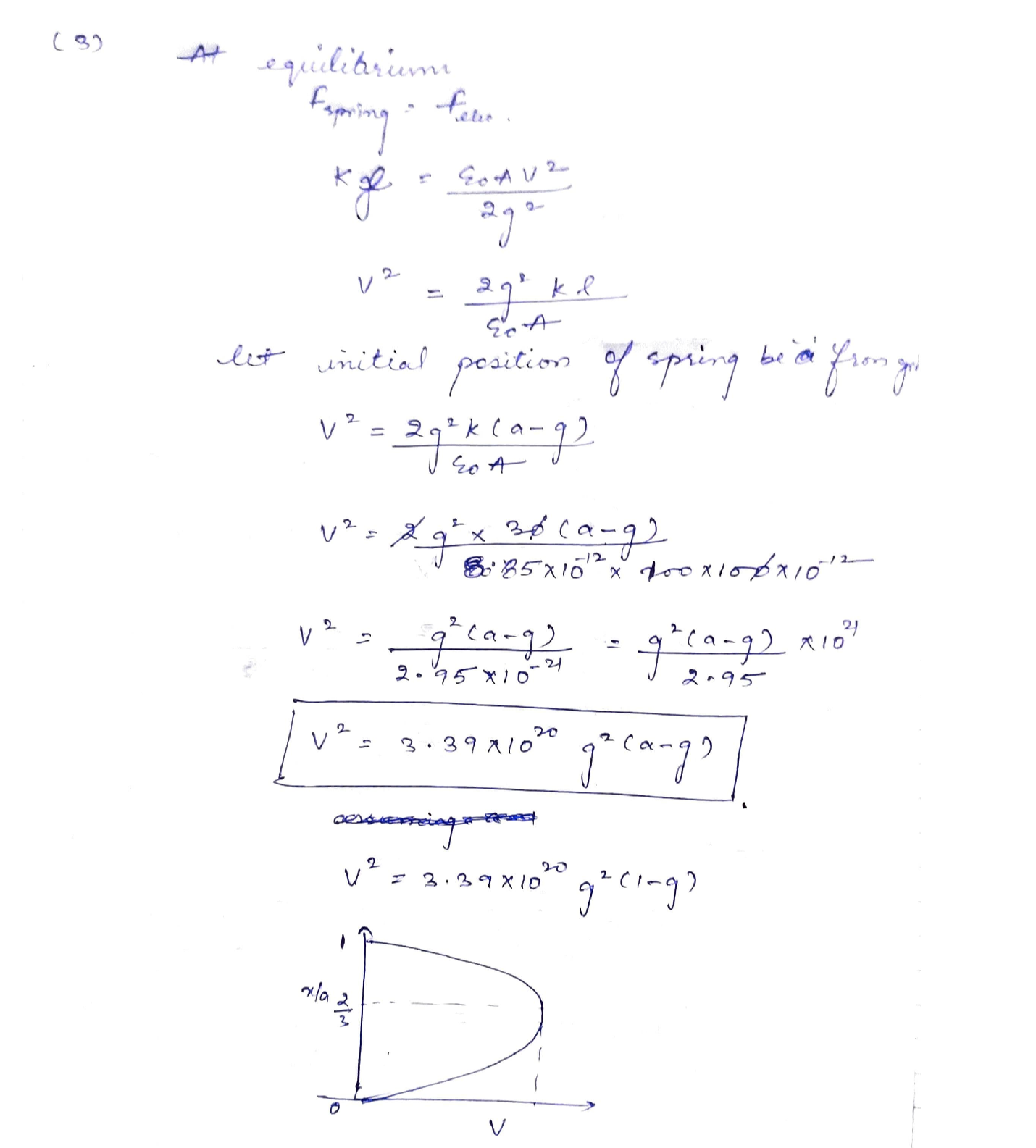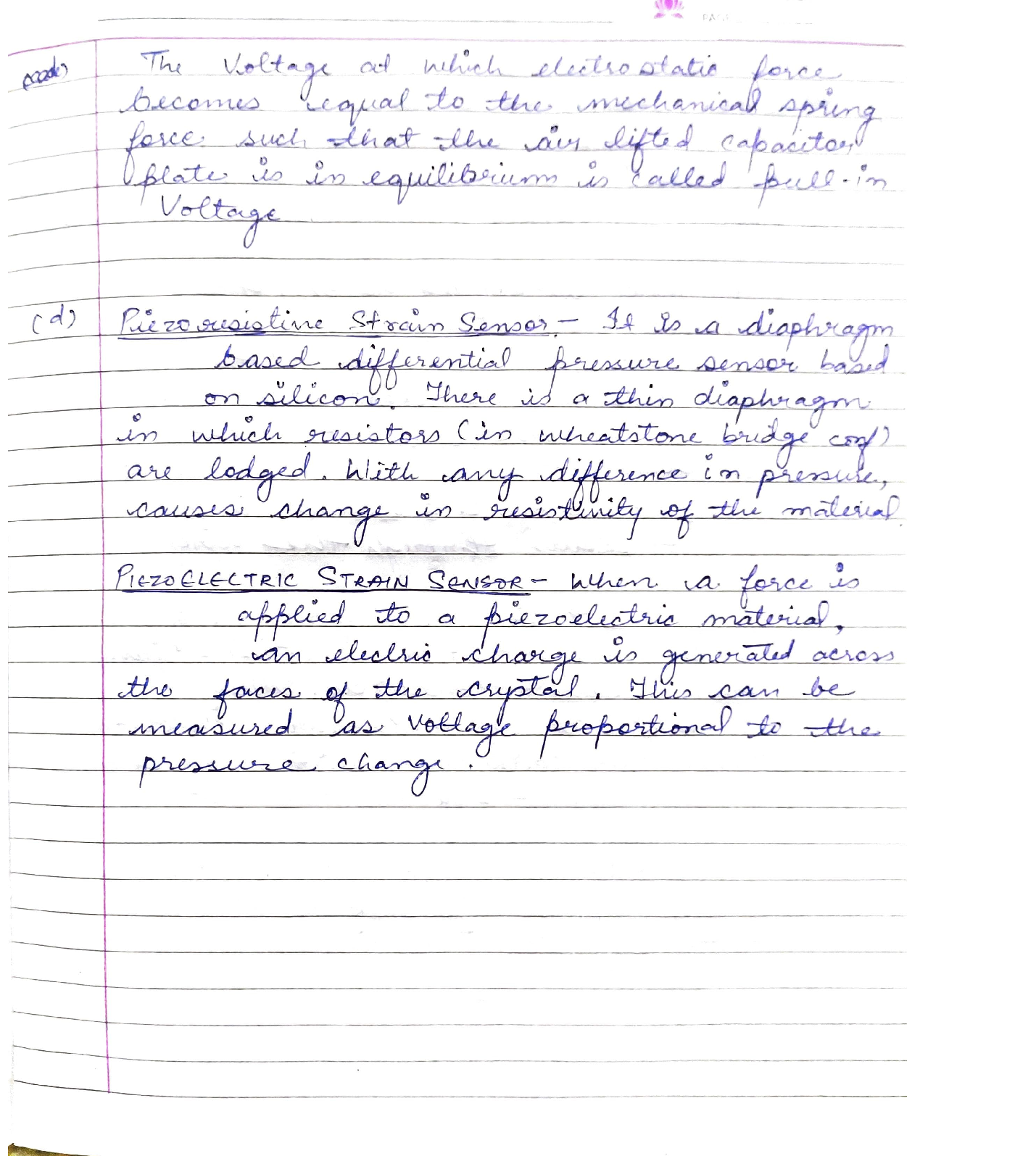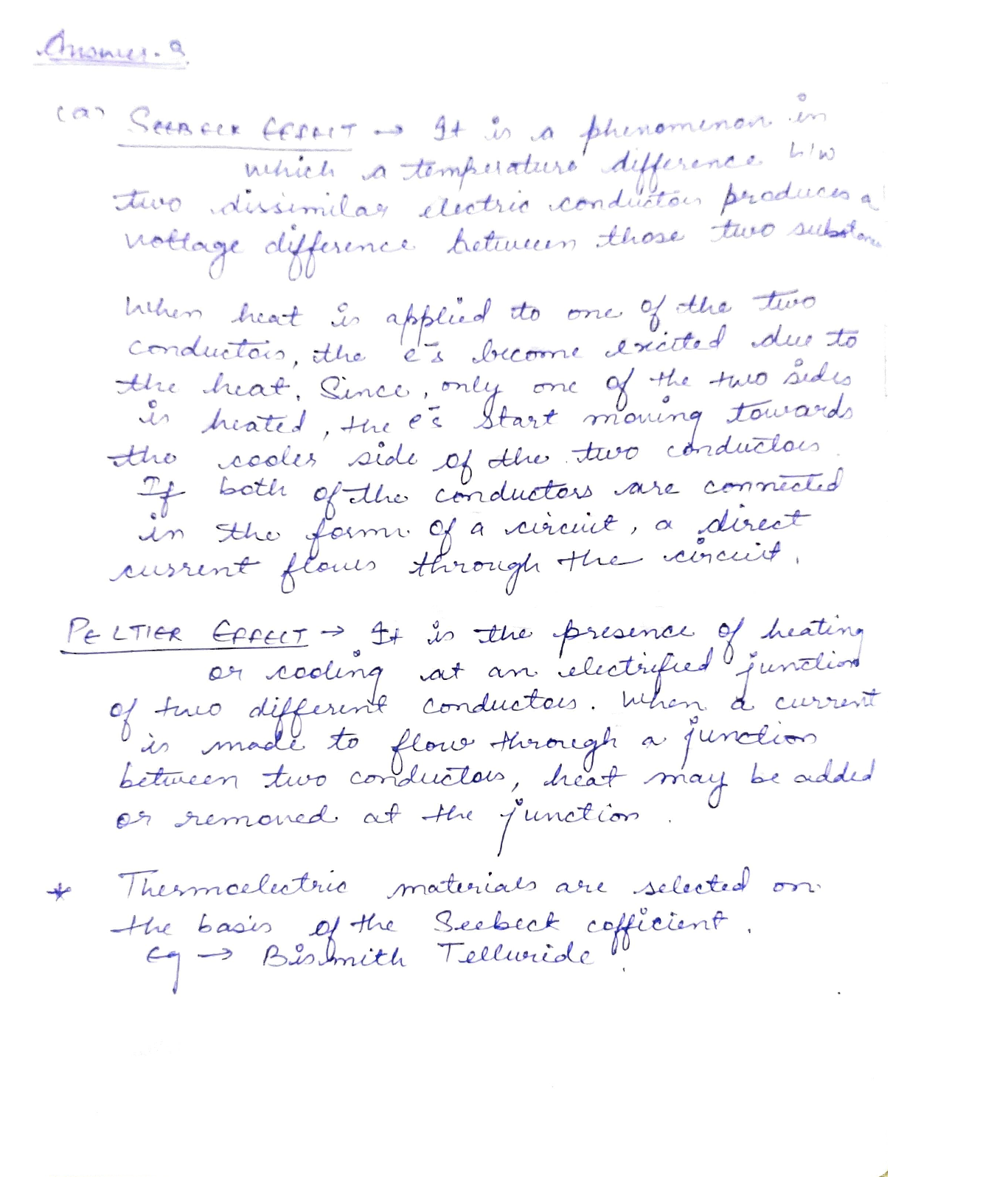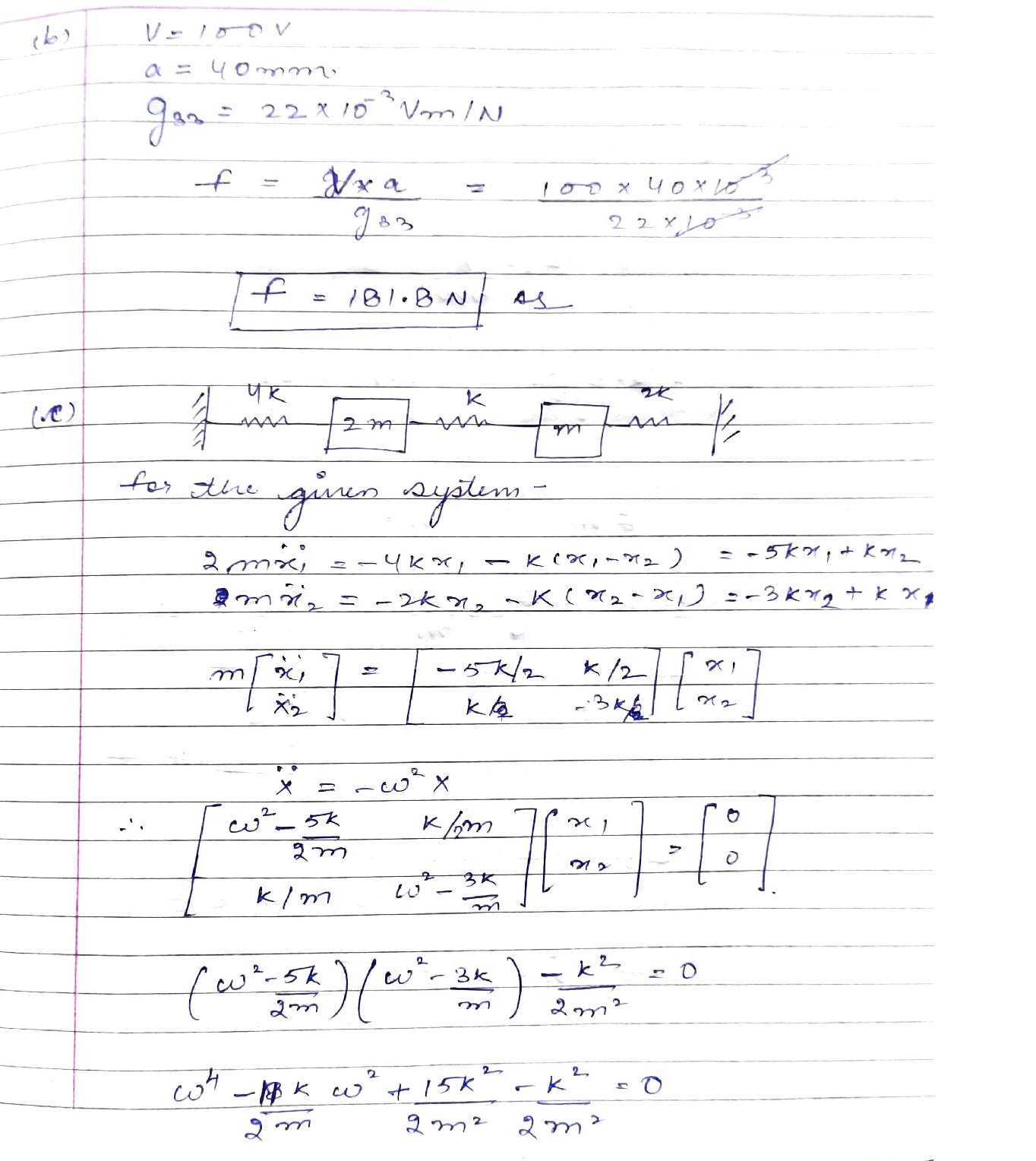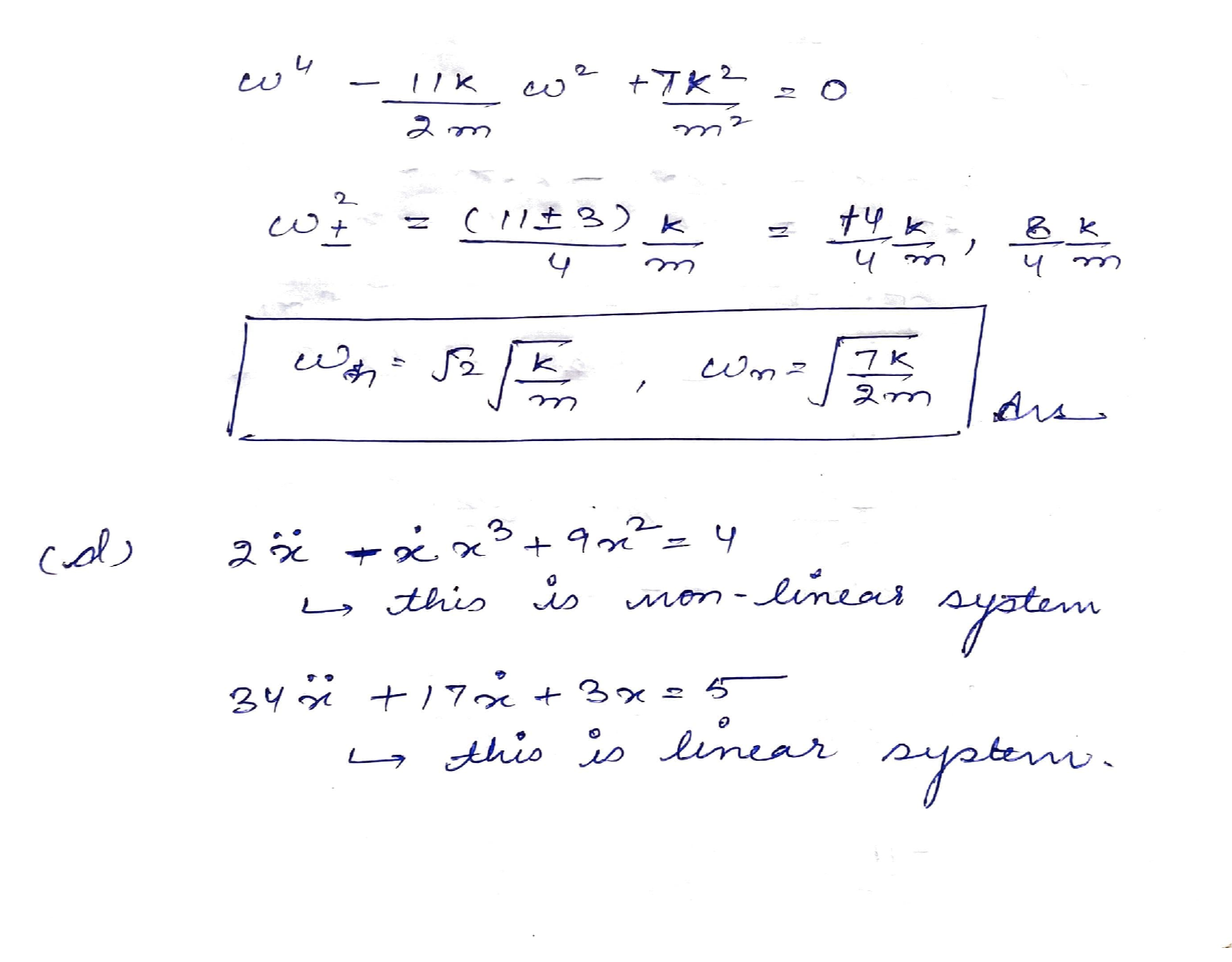### Question 45220Matlab And Simulation

6 Given the SSR
\dot{\mathrm{x}}=\left[\begin{array}{cc} -0.2 & -0.6 \\ 2 & -4 \end{array}\right] \mathrm{x}+\left[\begin{array}{c} 0 \\ 1.5 \end{array}\right] u \quad y=\left[\begin{array}{ll} 1 & 0 \end{array}\right] \mathbf{x}
Compute the eigenvalues "by hand."a.
b. Use MATLAB to verify your answer in part (a).
c. Describe the free response of the output y(t) given an arbitrary initial state x(0).
Use MATLAB or Simulink to verify your answer in part (c). The initial state vector is
\mathbf{x}(0)=\left[\begin{array}{ll} x_{1}(0) & x_{2}(0) \end{array}\right]^{T}=\left[\begin{array}{ll} -2 & -1 \end{array}\right]^{T}

### Question 45219Matlab And Simulation

15 Figure P7.15 shows a linear third-order system and a linear first-order system. Analytically and numericallyshow that the first-order transfer function G2(s) is an excellent low-order approximate model of the third-order system G,(8). Use MATLAB or Simulink for the numerical comparison.

### Question 40146Matlab And Simulation

(a) Use MATLAB ODE45 function to solve the below two second order differential equations (MATLAB scripts and calculated results/figures should be copied and pasted to the answer sheet). Plot x vs. t, i vs. t, and i vs. x,respectively.
\ddot{x}+15 \pi x+20 x^{3}=\sin (150 \pi t) ; \quad x_{0}=1 ; \dot{x}_{0}=1 ; \quad t \in
4 \ddot{x}+5 x \cdot \dot{x}+6 x=\sin \left(t^{2}\right) \quad x_{0}=2 ; \dot{x}_{0}=2 ; \quad t \in
(b) Describe the method of using the trajectory graph (velocity vs.displacement) to identify the stability of the system defined by a differential equation.
(c) In the motion equation of a MEMS (Micro-electromechanical systems)resonator, identify the factors affecting the natural frequency, and factors that contribute to the system damping. Write down the analytical equation of the fundamental natural frequency.
(d) Describe the procedure of fabricating a radio frequency (RF) MEMS switch with the top-down process, sketch out process flow.(6 mark)

### Question 40145Matlab And Simulation

(a) A DC voltage Vis applied to the microelectromechanical systems (MEMS)misaligned double-plate electrodes shown in Figure Q 1(a). The corresponding electrostatic force in Fd,direction is -epsilon0epsilonrWLV2)/ (2d2)(epsilon0 and epsilonr denote permittivity in vacuum and relative permittivity respectively. W, L, d are width, length, and gap between the two plates respectively). A linear scaling for the voltage is used, i.e. V x l',where I is the linear scale of the electrode. What happens to Fd if all dimensions of the two plates are reduced to 50% of their initial values?
(b) For the three differential equations below describing MEMS dynamic systems, write their transfer functions based on the Laplace Transform.Work out fundamental frequency for each case (neglect the damping effect).
3 \ddot{x}(t)+11 \dot{x}(t)+300 x=3 f(t)
a \dot{x}(t)+25 a x(t)=c f(t) ; \quad \text { a and } c \text { are non-zero constants }
\frac{1}{3} \ddot{x}(t)+27 x(t)=5 f(t)
(c) There is a parallel plate MEMS actuator shown in Figure Q 3(c). The initial gap go is 12 µm, and the area of the plate A is 200 µm x 100 µm. (Note:free space permittivity is 8.85 x 10-12 F/m)
(1) Derive the formula for the electrostatic force F;
(2) Assume a 5 volts potential is applied to the actuator. Calculate F when the top plate is in its initial position.
(3) Derive the equation for Vvs. g and draw a graph showing V vs. g (g varies from 0 to 12 um with 2 µm step size; given spring constant kis 30 N/m). Give a brief explanation about the pull-in voltage.
(d) Describe the working principle of a strain sensor that is made of a piezoresistive material and a piezoelectric material respectively.

### Question 34243Matlab And Simulation

1. Write MATLAB Live Script code (.mlx file) to estimate e for a user inputted value of x using the Taylor series expansion:
e^{x}=\sum_{n=0}^{\infty} \frac{x^{n}}{n !}=1+x+\frac{x^{2}}{2 !}+\frac{x^{3}}{3 !}+\frac{x^{4}}{4 !}+\ldots

### Question 34174Matlab And Simulation

3. [30 points] The following data gives the height (in inches) of a sunflower plant as a function of time(days after it was planted).
The height can be modeled by the logistic function:
H=\frac{100.8}{1+23 e^{-0.093 t}}
On the same figure, plot the height modeled by the equation as a solid blue line.
Where H is the height (in.) and t is the time (days). Make a plot of the height versus time. The figure should show the data from the table above as points only. Use red circles to plot each data point.
Add a legend in the southeast corner of your figure, labeling the data from the table as "Data Set" and the line from the equation as “Model". Include x and y labels for time and height. Make sure to include units in your labels. Include a title to your figure.

### Question 34173Matlab And Simulation

2. [20 points] Create the plot by using "Birth_Data.xlsx" excel data file which you can download from Problem set 3 Assignment in Blackboard. Make the code to input the data from excel (do not copy and paste the data or type the data in MATLAB).Add labels for each axis and legend (which needs to be making sense by understanding the excel data).Note: for excel file name, .xls would not work since the excel file is different version. Make sure to use.xlsx.

### Question 34172Matlab And Simulation

1. [48 points] Look at the following MATLAB script:
The code has numerous errors in it. It is supposed to compute the values of y = ax? + bx + c for various input parameters a, b, c, for x in the range of [s,e] (start to end) and save to a file called results.txt. Spot and correct the various errors. Your goal is to revise codes to run without error. Submit m. file with revised codes and use % to comment how you revised right above the revised coding.

### Question 32151Matlab And Simulation

Given the following open-loop plant, [Section: 12.2]
G(s)=\frac{50}{(s+1)(s+3)(s+10)}
design a controller to yield a 10% overshoot and a settling time of 0.5 second. Place the third pole10 times as far from the imaginary axis as the dominant pole pair. Use the phase variables for state-variable feedback.

### Question 29892Matlab And Simulation

Use the MATLAB code provided in the Radar Detection and Bernoulli Trial Application example given in class. Modify the detection threshold for multiple pulse returns; "m=round(n_iter/4)" to increase the number of threshold crossing events required for a detection. To do this modify this ton_iter/3. What happens to the probability of detection and probability of false alarm curves as compared to those associated with a threshold of n iter/4? Plot out the results for n iter/4 and also n iter/3.
Now try a threshold of n_iter/5 and record what changes occur to the probability of detection and probability of false alarm curves; again comparing to the results associated with the n_iter/4 threshold Can you explain the trends in both cases in terms of the Bernoulli trial equation?

### Submit query

Getting answers to your urgent problems is simple. Submit your query in the given box and get answers Instantly.

### Submit a new QuerySuccess

Assignment is successfully created